# PSAT 8/9 Math Practice Test 2022 (38 Questions and Answers)

PSAT 8/9 Math Practice Test 2022 (38 Questions and Answers): Try our free Preliminary SAT/National Merit Scholarship Qualifying Test review mathematics questions and answers. There are a total of 38 multiple-choice questions with answer keys and explanations. You can also download it as a printable pdf to use as a worksheet.

## PSAT 8/9 Math Practice Test 2022

 Test Name PSAT 8/9 Practice Test 2023 Sections Mathematics Total Items 38 MCQs Test Type Sample Online Test Time Limit NO Answer Keys/ Explanation Available

Q1. If y + 3 = 7, what is the value of 2y + 3 ?

• (A) –5
• (B) 8
• (C) 11
• (D) 14

The first step is to solve the first equation for y. To do that, subtract 3 from each side, yielding y = 4. Now plug that value into the second equation. This results in 2(4) + 3 = 8 + 3 = 11, so (C) is the credited response.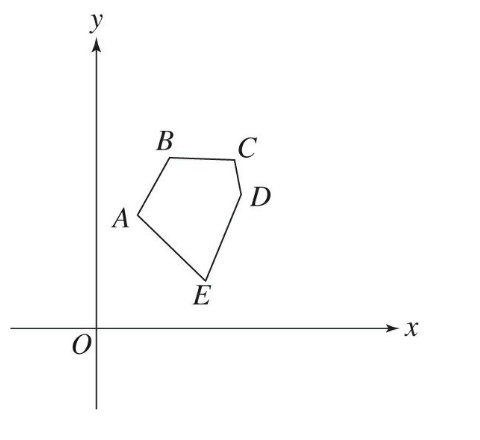Q2. In the figure above, which side of 5-sided polygon ABCDE has the greatest negative slope?

• (A) EA
• (B) AB
• (C) BC
• (D) CD

The question asks for the greatest negative slope, so the sides with positive slopes, AB and DE, can be eliminated. Side BC is practically a horizontal line; it has no slope and can also be eliminated. Of the two remaining sides, CD has the steepest slope, making (D) the credited response.

Q3. Rhys has a bucket of candy containing 15 lollipops, 25 gumballs, 35 licorice sticks, and no other types of candy. If Rhys selects one type of candy at random, what is the probability that the chosen candy will be a licorice stick?

• (A) \frac{1}{5}
• (B) \frac{1}{3}
• (C) \frac{3}{10}
• (D) \frac{7}{15}

Probability is defined as the number of things that fit the requirements divided by the total number of things. In this case, the number of licorice sticks would be divided by the total number of pieces of candy.

Q4. If (b + 2)(3b – 6) = z, then (b + 2)(b – 2) = ?

• (A) \frac{1}{6}z
• (B) 3x
• (C) \frac{1}{3}z
• (D) \frac{1}{2}z

With variables in the answer choices, the best option is to Plug In. Choose an easy number for b such as 4. This makes the first equation (4 + 2)(3(4) – 6), which equals (6)(6). Therefore z = 36. The second equation is (4 + 2)(4 – 2), which equals (6)(2) or 12. This is the target answer.

Q5. If three lines intersect to form six equal angles, what is the measure of one of these angles?

• (A) 15°
• (B) 30°
• (C) 45°
• (D) 60°

There are 360° in a plane. If three lines meet a plane to form 6 equal angles, the lines will divide the total 360° by 6, leaving 60° per angle. Therefore, (D) is the credited response.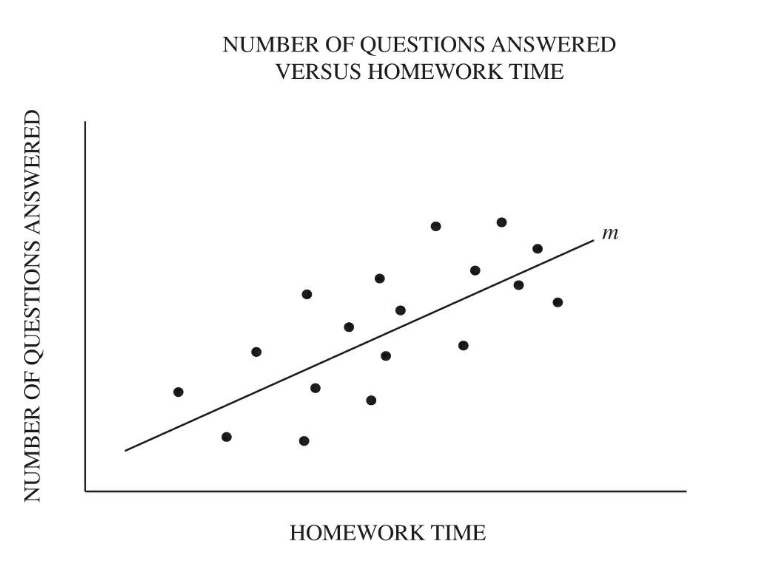Q6. Brian surveyed the 18 students in his algebra class to determine the amount of time they spent doing homework and the number of questions answered on the last test. The scatterplot above shows the homework time and the number of test questions answered for each of the 18 students. Line m is the best fit line. For how many students was the number of test questions answered fewer than what the best fit line predicted for the corresponding homework time?

• (A) 8
• (B) 9
• (C) 10
• (D) 11

Each dot on the scatterplot represents one student. To find the number of students who answered fewer questions than expected, count the number of dots below the best fit line. There are 8, so (A) is the credited response.

Q7. The expression 3p + 6 could be represented by which of the following operations?

• (A) 3 times 6 more than a number p
• (B) 3 times 6 less than a number p
• (C) 3 more than 6 times a number p
• (D) 6 more than 3 times a number p

Q8. If the quantity (2t – 5) is divisible by t, which of the following could be the value of t ?

• (A) 4
• (B) 5
• (C) 6
• (D) 7

When a question asks for a specific amount and the answer choices are numbers, Plug in the Answers (PITA).  With PITA, only one answer choice will work, so there is no need to try the others.

Q9. In the figure above, AD = DB = EC = CF. If ΔABC and ΔDEF are equilateral, and AB = 10, what is the perimeter of quadrilateral CGDH?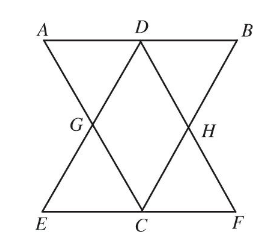• (A) 10
• (B) 15
• (C) 20
• (D) 25
• (E) 35

Start by marking all the information given in the problem on the figure. If ∆ABC and ∆DEF are equilateral, then all the long sides are 10, and AD = DB = EC = CF = 5. Because C and D are midpoints of AB and EF, those lines are parallel. This means that all the small triangles are also equilateral triangles, with side lengths of 5. If each side of quadrilateral CGDH is 5, the perimeter is 20, making (C) the credited response..

Q10. Helene lists her zip code on an application form. She notices that the first and last digits are both prime, the middle digit is a divisor of 45, and the least common multiple of the remaining two digits is 6. Which of the following numbers could be her zip code?

• (A) 22935
• (B) 23924
• (C) 32437
• (D) 35563

Use the process of elimination on the answer choices. The first digits are all prime numbers, so no answers can be eliminated from that.

Q11. What number is 4 less than \frac{1}{2} of itself?

• (A) –11
• (B) –10
• (C) –9
• (D) –8

When the question asks for a specific number, use PITA. The first step is taking half of the number, which won’t come out evenly with odd numbers.

Q12. Ringo is a dog that is not brown. From which of the following statements can it be determined whether or not Ringo is a Labrador?

• (A) Any dog that is not brown is not a Labrador.
• (B) Some dogs that are brown are not Labradors.
• (C) All dogs that are brown are Labradors.
• (D) No dogs are both brown and Labradors.

This is a logic question, so check each answer to determine if it helps provide more necessary information about Ringo.

Q13. The side of square S is twice the diameter of circle C. What is the greatest possible number of points of intersection of the perimeter of square S and the circumference of circle C ?

• (A) Two
• (B) Three
• (C) Four
• (D) Six

To determine the possible number of points of intersection between two shapes, draw them a few different ways. Move the shapes around and try to overlap them as much as possible.

Q14. If the ordered pair (t, 2), where x = t and y = 2, is the intersection of the two lines above, then what is the value of n ?

y = –x
y = x + n

• (A) –2
• (B) 0
• (C) 2
• (D) 4

This question has a lot of variables, so start by plugging in the given information. The language about the ordered pair is confusing, but it clearly states “x = t and y = 2.” Plug those values in to the two equations,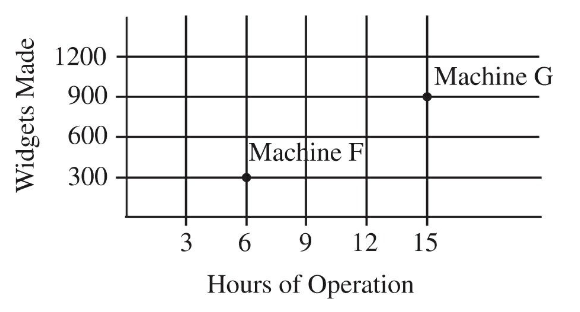Q15. The graph above shows the number of widgets made by two machines, F and G, plotted against the number of hours in operation. On average, for each hour of operation, how many more widgets did machine G make than machine F?

• (A) 9
• (B) 10
• (C) 22
• (D) 50

To answer this question, the number of widgets each machine makes in one hour must be calculated. According to the chart, Machine F makes 300 widgets in 6 hours of operation. Divide 300 by 6 to get 50 widgets per hour for machine F. Machine G makes 900 widgets in 15 hours of operation. Divide 900 by 15 to get 60 widgets per hour for machine G. To find the difference in number of widgets made for each hour of operation, subtract 50 from 60, which equals 10. Therefore, (B) is the credited response.

Q16. If w is an integer and 8 is a factor of 2w, which of the following must be true?

I. w is an even integer
II. w is divisible by 4
III. w is divisible by 8

• (A) I only
• (B) II only
• (C) I and II only
• (D) II and III only
• (E) I, II, and III

Q17. If q and s are greater than 0 and \frac{q¹²}{q²} = s5, then = \frac{s¹²}{s²}

• (A) q5
• (B) q6
• (C) q10
• (D) q20

Q18. Line l, defined by the equation x + 2y = 4, is graphed in the xy-plane. Line m is the reflection of line l across the x-axis. Which is the following is an equation of line m ?

• (A) x – 2y = –4
• (B) x + 2y = –4
• (C) x – 2y = 4
• (D) x + 2y = 4

Q19. A lock requires a 3-digit code such that each digit is a positive even number less than 9. How many such codes are possible?

• (A) 4
• (B) 8
• (C) 16
• (D) 64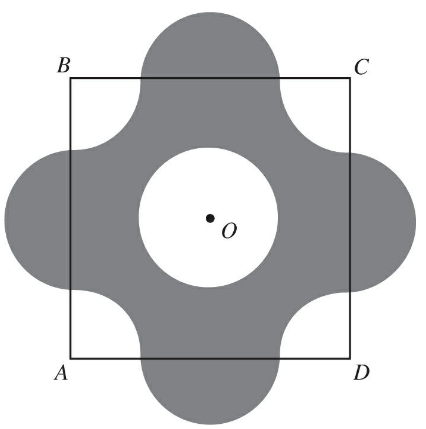Q20. The figure above shows a square with side of length 4. The center of the square, point O, is also the center of circle O, with radius 1. Four semicircles with radius 1 are centered on each side of the square. If the arcs shown have centers at A, B, C, and D, what is the area of the shaded region?

• (A) 4π
• (B) 16
• (C) 8π – 8
• (D) 8 + 4π

Q21. If r is an integer and 7.3 × 10r is a number between 8,000 and 80,000, what is the value of r ?

• (A) 1
• (B) 2
• (C) 3
• (D) 4

Q22. In the xy-plane above, ABCD is a square with a side length of 8. If line m passes through points A and C, which of the following points lies on line m ?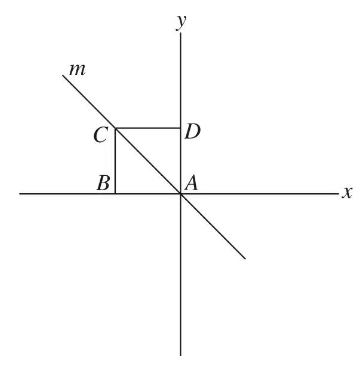• (A) (–5, 0)
• (B) (0, –5)
• (C) (–5, 5)
• (D) (0, 5)

Q23. A total of 30,000 students attend College Q. How many of the students have a GPA that is less than 4.0 ?

• (A) 3,000
• (B) 6,000
• (C) 12,000
• (D) 24,000

3, 15, 35, 63, …

Q24. The sequence above is formed by multiplying each odd integer by the next odd integer, starting with 1. What is the difference between the 7th and 8th term of the sequence?

• (A) 1
• (B) 28
• (C) 60
• (D) 128

Q25. When Gilbert rents a car from the Cars4U dealership, he is charged a constant rate per mile the car is driven plus a fixed cost for a carwash. On Monday he was charged a total of $13 after driving the rental car for 20 miles. On Friday he was charged$11.50 for driving the rental car 14 miles. Which of the following equations expresses the total charge T, in dollars, when Gilbert drives the rental car for m miles?

• (A) T = m – 7
• (B) T = 0.25m
• (C) T = 0.25m + 8
• (D) T = 0.5m + 4

Q26. The function g is defined by g(x) = 33 – 4x. If g(b) = 5, what is the value of b ?

• (A) \frac{19}{2}
• (B) -7
• (C) 7
• (D) \frac{19}{2}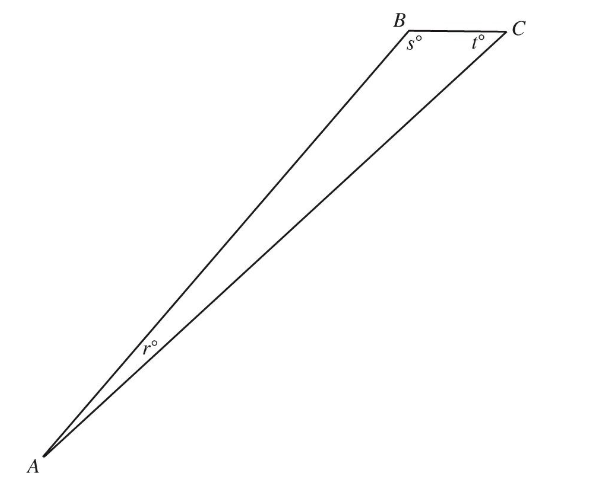Q27. In the figure above, AB = 200 and BC = 5. Which of the following must be true?

• (A) r is less than s and t
• (B) s is greater than r and t
• (C) t is greater than r and s
• (D) s is equal to t

Q28. At a certain department store, the only sizes of shirts sold are small, medium, and large. At that store, the ratio of small shirts to medium shirts is 5:2, and the ratio of medium shirts to large shirts is 6:5. What is the ratio of small shirts to all other shirts?

• (A) 5:11
• (B) 5:16
• (C) 7:11
• (D) 5:11

Q29. Each of the 8 salesmen at a used car dealership met his sales quota of 5 cars last month. In addition, 3 of the salesmen each sold exactly 3 additional cars, and 2 of the salesmen each sold 1 additional car. What is the total number of cars sold by these 8 salesmen?

• (A) 11
• (B) 21
• (C) 41
• (D) 51

Q30. In 1976 the world record distance for throwing a discus was 230 feet, and by 1983 the record distance had increased to 235 feet and 3 inches. If the record distance continues to increase at the same rate, in what year will the record distance for throwing a discus reach 261 \frac{1}{2} feet?

• (A) 18
• (B) 118
• (C) 1,018
• (D) 2,018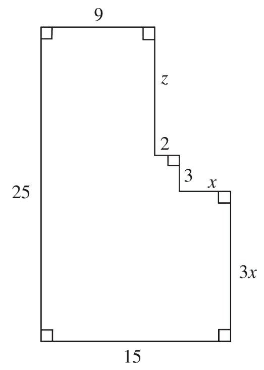Q31. In the figure above, what is the value of z ?

• (A) 1
• (B) 10
• (C) 11
• (D) 110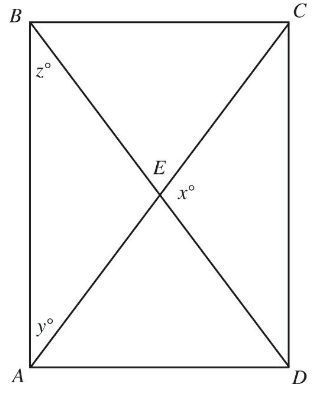Q32. In the figure above, AC and BD are the diagonals of rectangle ABCD. If x = 123, what is the value of z ?

• (A) 28.5
• (B) 25.5
• (C) 38.5
• (D) 48.5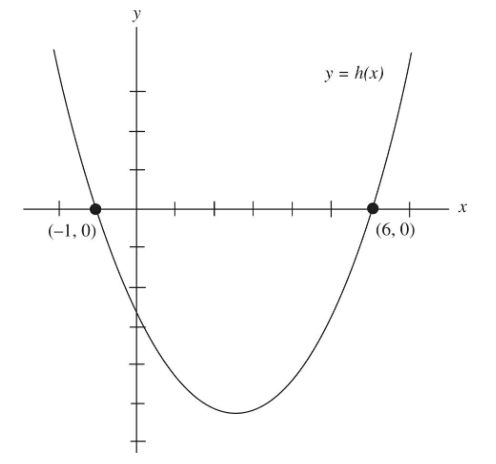Q33. In the graph above, h(x) is a quadratic function. For what x value does h(x) reach its least value?

• (A) 1.5
• (B) 2.5
• (C) 6.5
• (D) 12.5

Q34. On her first four quizzes, Jan score 12, 8, 1, and 15 points. After Jan took her fifth quiz, she found that the average (arithmetic mean) of the five quiz scores was equal to the median of the five quiz scores. What is one possible value for the number of points Jan scored on her fifth quiz?

• (A) 2, 3, 8
• (B) 1, 9, 14
• (C) 3, 3, 21
• (D) 4, 9, 24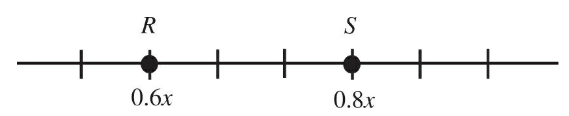Q35. On the number line above, the distance between point R and point S is 3. What is the value of x?

• (A) 3
• (B) 15
• (C) 25
• (D) 35

Q36. Sara created a list of 500 consecutive multiples of 4. What is the result if Sara subtracts the smallest number in her list from the largest number her the list?

• (A) 1233
• (B) 1195
• (C) 1996
• (D) 3596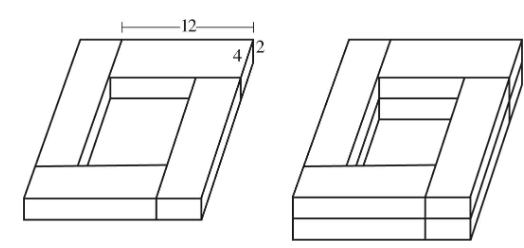Q37. Four boards each measuring 2 inches by 4 inches by 12 inches are laid on a flat floor to form a square, as shown in the figure on the left. Another four boards of the same dimensions are laid on top of the first four in the same way, as shown in the figure on the right. This process is repeated until the boards are stacked a total of 10 boards high. The box created by these boards is then filled with sand until the sand is perfectly level with the highest point of the box. What is the volume, in cubic inches, of the sand in the box?

• (A) 1,280
• (B) 1,490
• (C) 1,690
• (D) 1,990